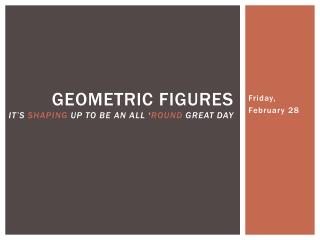Download PresentationGeometric Figures It’s shaping up to be an all ‘ round great day

# Geometric Figures It’s shaping up to be an all ‘ round great day

Download Presentation## Geometric Figures It’s shaping up to be an all ‘ round great day

- - - - - - - - - - - - - - - - - - - - - - - - - - - E N D - - - - - - - - - - - - - - - - - - - - - - - - - - -
##### Presentation Transcript

1. Geometric FiguresIt’s shaping up to be an all ‘round great day Friday, February 28

2. Wayward Whyday • Because we missed Wednesday this week… Why does a charged balloon stick to a wall?

3. Investigative activity #1 • Pick any three points and draw a triangle ABC • Find the midpoints of the lines AB and AC. • Draw a line between those midpoints. • Compare the length of the line between the midpoints to the length of the line BC • Compare the slope of the line between the midpoints to the slope of the line BC B A C

4. Geometric properties of triangles B A C The line between these midpoints is parallel to BC The length of the line between these midpoints is half the length of BC

5. Investigative activity #2 • Draw a circle with a radius of 5. • Draw any chord through the circle (that’s a line that connects two points on the circle but doesn’t go through the center point) • Calculate the midpoint of the chord. • Determine the slope of a line perpendicular to the chord. • Draw a line starting at the midpoint of the chord, running perpendicular to the chord. • What do you notice about how this perpendicular line is related to the center of the circle? chord

6. Investigative activity #2 The perpendicular bisector of any chord in a circle (that’s the line that runs perpendicular to and through the midpoint of a chord) also goes through the center of the circle. chord perpendicular bisector

7. Investigative activity #3 • Draw any triangle on the graph paper. • Calculate the midpoint on one of the three sides of your triangle. • Calculate the slope of a line perpendicular to the side you chose. • Draw the perpendicular bisector to the side you chose. • Repeat steps 2 – 4 for the other two sides. • What do you notice about these three perpendicular bisectors?

8. The circumcenter The circumcenter is the point where the perpendicular bisectors coming from each of the three sides of a triangle intersect. Awesomely enough, it’s also the center of a circle drawn around the triangle!

9. Investigative activity #4 • Draw any triangle on the graph paper. • Calculate the midpoint on one of the three sides of your triangle. • Draw lines from these midpoints to the opposite corner of the triangle. These lines are called “medians”. • Repeat steps 2 – 3 for the other two sides. • What do you notice about these three lines?

10. The Centroid The lines joining the midpoints of each side to the opposite corner are called medians. The centroid is the intersection of the medians of a triangle.

11. Applying slope to geometry Michelle Krissy Amy • Create a diagram of the baseball diamond with coordinates of each point (#1) Erica Natasha Braden • Create a infographic showing how you calculated the slopes (#2) Everyone work through the activity on page 88 James B Ben Laura • Create an infographic about perpendicular slopes (#3) James J Jarrod Bryce Katelyn • Create a infographic about midpoints and distances (#4 and 5)

12. Homework • Page 95 #1, 2, 5, 21